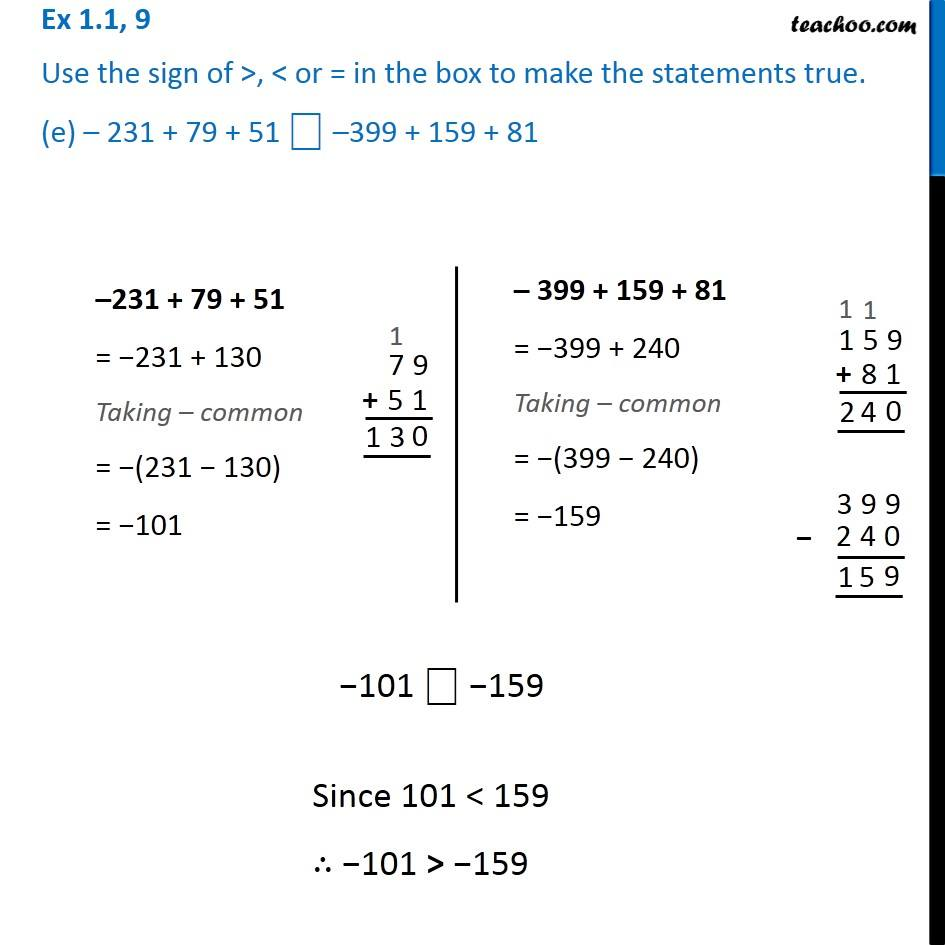Chapter 1 Class 7 Integers
Serial order wiseLearn in your speed, with individual attention - Teachoo Maths 1-on-1 Class

### Transcript

Question 9 Use the sign of >, < or = in the box to make the statements true. (e) – 231 + 79 + 51 ⎕ –399 + 159 + 8139 + (– 24) – (15) ⎕ 36 + (– 52) – (– 36) 39 + (– 24) – (15) = 39 − 24 − 15 Taking – common = 39 − (24 + 15) = 39 − 39 = 0 36 + (– 52) – (– 36) = 36 − 52 + 36 = 36 + 36 − 52 = 72 − 52 = 20 0 ⎕ 20 0 < 20 Question 9 Use the sign of >, < or = in the box to make the statements true. (e) – 231 + 79 + 51 ⎕ –399 + 159 + 81–231 + 79 + 51 = −231 + 130 Taking – common = −(231 − 130) = −101 – 399 + 159 + 81 = −399 + 240 Taking – common = −(399 − 240) = −159 −101 ⎕ −159 −101 ⎕ −159